Gnuplot, GLPK
gnuplotglpk
version used

5.04.52
show version

$gnuplot --version$ glpsol --version
grammar and invocation
gnuplotglpk
interpreter

$cat hello.gnuplot print "Hello, World!"$ gnuplot hello.gnuplot
Hello, World!
$cat hello.model printf "Hello, World!\n"; end;$ glpsol -m hello.model
Hello, World!
repl

$gnuplotnone compilernonenone command line program$ gnuplot -e 'print 1 + 1;'none
block delimiters

{ }{ }

Curly braces are used to delimit the block of statements in the body of a for statement.

Curly braces are also used in indexing expressions and set literals.
statement separatornewline or ;

A newline preceded by a backslash is ignored.
;
end-of-line comment

# comment# comment
multiple line comment

none/* comment line
another comment */
variables and expressions
gnuplotglpk
assignmentx = 3.14# parameters are write once:
param pi := 3.14;
null

nonenone
null test

nonenone
undefined variable access

causes errorcauses error
undefined variable testexists("x")none
remove variable bindingundefine xnone
conditional expression

x > 0 : x : -xif x > 0 then x else -x
arithmetic and logic
gnuplotglpk
true and false

1 01 0

The boolean values are distinct from the integers 1 and 0. When displayed by printf '%s' they render as T and F. There are no boolean literals, however.
falsehoods

0

0.0 is true
0 0.0
logical operators&& || !and or not

&& || !
relational operators== != < > <= >=

# string comparision:
eq ne
= <> < > <= >=

== and != are synonyms of = and <>
arithmetic operators

addition, subtraction, multiplication, division, modulus
+ - * / %+ - * / mod
integer division

int(x) / int(y)x div y
integer division by zeroerrorerror
float division(1.0 * x) / y7 / 3
float division by zeroerrorerror
power2 ** 322 ^ 32
2 ** 32
sqrtsqrt(2)sqrt(2)
sqrt -1

# evaluates to {0.0, 1.0}:
sqrt(-1)
# error:
sqrt(-1)
transcendental functionsexp() log log10()
sin() cos() tan()
asin() acos() atan()
atan2()
exp() log() log10()
sin() cos() none
none none atan()
atan(y, x)
transcendental constants
e and pi
exp(1)
pi
exp(1)
4 * atan(1)
float truncation
round towards zero, round to nearest integer, round down, round up
int(x)
??
floor(x)
ceil(x)
trunc(x)
round(x)
floor(x)
ceil(x)

# truncate/round to 2 decimal places:
trunc(x, 2)
round(x, 2)
absolute value
and signum
abs(x)
sgn(x)
abs(x)
none
integer overflow

32 bit signed integers are used. Operations which overflow return a float.conversion to float
float overflow

64 bit double precision floats are used. Operations which overflow raise an error.error
complex construction

z = {1.0, 2.0}none
complex decomposition
real and imaginary part, argument and modulus, conjugate
real(z)
imag(z)
arg(z)
abs(z)
??
none
random number
uniform integer, uniform float, standard normal
floor(rand(0) * 100)
rand(0)
none
# Irand224() generates integer in [0, 2^24):
Irand224() mod 100
Uniform01()
Normal01()
random seed_ = rand(17)$glpsol --seed 17 -m foo.model bit operators << >> & | ^ ~none binary, octal, and hex literalsnone 052 0x2a none strings gnuplotglpk string literals"don't say \"no\"" 'don''t say "no"' "don't say ""no""" 'don''t say "no"' Quotes can be omitted in the data section when the contents of the string are alphanumeric or match the character class [-+_.] newline in literalnono string literal escapes# in double quotes: \n \r \t \o \oo \ooo # in printf format: \n \t lengthstrlen("lorem ipsum")length("lorem ipsum") concatenate"lorem " . "ipsum""lorem " & "ipsum" index of substring# indices start at 1; # returns 0 if not found: # strstrt("foo bar", "bar", none extract substringsubstr("foo bar", 5, 8)substr("foo bar", 5, 3) format stringsprintf("%d %.2f %s", 7, 3.1415, "foo")none number to string"foo" . 4none string to number"4" + 7 # causes error: "foo" + 7 none dates and time gnuplotglpk current time as unix epoch# seconds since Jan 1, 2000 # add 946684800.0 to get unix epoch: now = time(0) # as float: real_now = time(0.0) gmtime() format timestrftime('%Y-%m-%d %H:%M:%S', now)time2str(gmtime(), '%Y-%m-%d %H:%M:%S') parse times = "2014-11-30 03:53:59" t = strptime('%Y-%m-%d %H:%M:%S', s) str2time("2014-12-01 19:38:09", "%Y-%m-%d %H:%M:%S") sleeppause 10 # with message: pause 10 'sleeping 10s...' none multidimensional arrays gnuplotglpk 2d literalin separate file; one line for each data point; whitespace delimited:$ cat data.txt
7 8
9 10
11 12
# declare in model section:
param a {1..2, 1..2};

# define in data section:
param a: 1  2 :=
1  7  8
2  9 10;
3d literalin separate file; one line for each data point:

$cat data.txt # city data: "Los Angeles" 3 7 "New York" 8 4 "San Francisco" 12 1 comment lines are ignored; data which contains whitespace must be quoted sets gnuplotglpk literalset digits := {1, 2, 3, 4, 5}; set from arithmetic sequence unit difference, non-unit difference set digits := {1 .. 5}; set leap_years := {2000 .. 2096 by 4}; membershipset s := {1, 2, 3}; set str := if 4 in s then 'yes' else 'no'; intersectionset s1 := {1, 2, 3}; set s2 := {2, 3, 4, 5}; set s3 := s1 inter s2; unionset s3 := s1 union s2; relative complement, symmetric differenceset s3 := s1 diff s2; set s4 := s1 symdiff s2; cartesian product# 12 pairs: set s3 := s1 cross s2; mapset s := 1 .. 10; set squares := setof {i in s} i * i; reduceset s := 1 .. 10; param n1 := min {i in s} i; # 1 param n2 := max {i in s} i; # 10 param n3 := sum {i in s} i; # 55 param n4 := prod {i in s} i; # 10! # sum of squares: param n5 := sum {i in s} i * i; universal testset str1 := if (forall {i in s} i > 5) then 'yes' else 'no'; existential testset str2 = if (exists {i in s} i > 5) then 'yes' else 'no'; functions gnuplotglpk define function# body of function must be an expression: add(x, y) = x + y none invoke functionadd(3, 7)none list functionsshow functionsnone missing argument behaviorerrornone extra argument behaviorerrornone return valuevalue of the expression which is the function bodynone execution control gnuplotglpk ifif (x > 0) { print "positive" } else { if (x < 0) { print "negative" } else { print "zero" } } none whilei = 0 while (i < 10) { print i i = i + 1 } none fordo for [i = 1:10] { print i } for {i in {1..10}} { printf "%d\n", i; } break continue raise exception file handles gnuplotglpk write line to stdoutprint "Hello, World!"printf "Hello, World!\n"; write formatted string to stdoutprintf "%d %.2f %s", 7, 3.1415, 'foo'; write to file# overwrites contents of /tmp/foo.txt: printf "lorem ispum" > "/tmp/foo.txt"; append to fileprintf "dolor sit amet" >> "/tmp/foo.txt"; pretty printset s := {1, 2, 3, 4, 5}; param x := 3; param str symbolic := 'lorem ispum'; display "set: ", s, "param: ", x, "string: ", str; directories gnuplotglpk working directory# writes working dir to stdout: pwd cd "/tmp" none libraries and namespaces gnuplotglpk load$ cat foo.gnuplot
print "loaded!"

$cat main.gnuplot load 'foo.gnuplot'$ gnuplot main.gnuplot
loaded!
none
processes and environment
gnuplotglpk
command line arguments$cat test.gnuplot print foo print bar$ gnuplot -e 'foo=3; bar=4' test.gnuplot
none
exit# no way to set exit status:
exit

# or:
quit
none
command substitutionls_output = system("ls")none
optimization
gnuplotglpk
linear minimizationvar x1;
var x2;
var x3;

minimize obj: x1 + x2 + x3;

c1: x1 + x2 >= 1;
c2: x2 + x3 >= 1;
c3: x1 + x3 >= 1;

solve;
display obj, x1, x2, x3;
end;
decision variable arrayset I := {1 .. 3};
var x{I};

minimize obj: sum{i in I} x[i];

c1: x + x >= 1;
c2: x + x >= 1;
c3: x + x >= 1;

solve;
display obj, x;
end;
linear maximizationset I := {1 .. 3};
var x{I};

maximize obj: sum{i in I} x[i];

c1: x + x <= 1;
c2: x + x <= 1;
c3: x + x <= 1;

solve;
display obj, x;
end;
constraint in variable declarationset I := {1 .. 3};
var x{I} >= 0;

minimize obj: sum{i in I} x[i];

c1: -x + x >= 1;
c2: x + x >= 1;
c3: -x + x >= 1;

solve;
display obj, x;
end;
unbounded behavior

# Stops execution after solve statement with "LP HAS UNBOUNDED PRIMAL SOLUTION" message.
infeasible behavior# Stops execution after solve statement with "LP HAS NO PRIMAL FEASIBLE SOLUTION" message.
integer decision variableset I := {1 .. 3};
var x{I} integer;

minimize obj: sum{i in I} x[i];

c1: x + x >= 1;
c2: x + x >= 1;
c3: x + x >= 1;

solve;
display obj, x;
end;
binary decision variableset I := {1 .. 3};
var x{I} binary;

minimize obj: sum{i in I} x[i];

c1: x + x >= 1;
c2: x + x >= 1;
c3: x + x >= 1;

solve;
display obj, x;
end;
linear regression
gnuplotglpk
bar charts
gnuplotglpk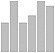vertical bar chart
# echo $'7\n3\n8\n5\n5' > data.txt set terminal png size 400,300 set output "barchart.png" set boxwidth 0.9 set style fill solid 1.0 plot [0:6] [0:9] "data.txt" \ using 0:1 notitle with boxes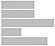horizontal bar chart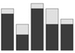stacked bar chart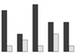grouped bar chartpie chart see note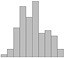histogram # awk '{ print length($0)}' /usr/share/dict/words > hist.txt

set terminal png size 400,300
set output "histogram.png"
binwidth=1
bin(x,width)=width*floor(x/width)
unset key
plot "hist.txt" \
using (bin(\$1,binwidth)):(1.0) \
smooth freq with boxes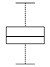box plot
# seq 100 | awk '{print rand() ** 3}' > data.txt

set terminal png size 400,300
set output "boxplot.png"
set style boxplot outliers pointtype 7
set style data boxplot
set boxwidth 0.2
plot [0.5:1.5] [0.0:1.1] "data.txt" \
using (1.0):1:(0.3) notitle
scatter plots
gnuplotglpk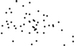scatter plot
# seq 50 | awk '{x=rand(); print x,x+rand()}' > data.txt

set terminal png size 400,300
set output "scatter.png"
unset key
plot "data.txt"
bubble chart
3d scatter plot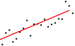linear regression line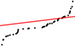quantile-quantile plot
line and surface charts
gnuplotglpk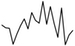polygonal line plot
# seq 1 20 | awk '{print rand() + rand() + rand()}' > data.txt

set terminal png size 400,300
set output "polygonal.png"
unset key
plot "data.txt" using 0:1 with lines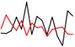additional line
# seq 1 20 | awk '{print rand() + rand(), rand() + rand()}' \
#   > data.txt

set terminal png size 400,300
set output "two-lines.png"
unset key
plot "data.txt" using 0:1 with lines, \
"data.txt" using 0:2 with lines
line typescubic splinefunction plot
set terminal png size 400,300
set output 'sin.png'
unset key
plot [-4:4] sin(x)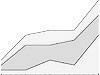area chart
surface plot
contour plot
chart-options
gnuplotglpk
set chart titleset title "a plot"
plot "data.txt"
axis label
axis limits
logarithmic y-axis
legend# seq 1 20 | awk '{print rand() + rand(), rand() + rand()}' \
#   > data.txt

set terminal png size 400,300
set output "legend.png"
plot "data.txt" using 0:1 with lines title "first", \
"data.txt" using 0:2 with lines title "second"
legend options
colors# use a string to specify a color:
set terminal png background "black"
set terminal png background "#000000"

# list recognized color names:
show colors
______________________________________________________________________________________________________________________________________

# General

## version used

The version used to test examples in this sheet.

## show version

How to determine the version.

# Grammar and Invocation

## interpreter

How to execute a script.

pari/gp

The shebang style notation doesn't work because GP doesn't recognize the hash tag # as the start of a comment.

The -q option suppresses the GP startup message.

After the script finishes it will drop the user into the REPL unless there is a quit statement in the script:

print("Hello, World!")

quit


# Bar Charts

## pie chart

gnuplot:

set terminal png size 400,400
set output "piechart.png"
set style fill solid 1.0 border -1

tot = 7 + 3 + 8 + 5 + 5
angle1 = 7.0 / tot * 360
angle2 = (7.0 + 3) / tot * 360
angle3 = (7.0 + 3 + 8) / tot * 360
angle4 = (7.0 + 3 + 8 + 5) / tot * 360

set object 1 circle at screen 0.5,0.5 size \
screen 0.45 arc [0:angle1] fillcolor rgb "#FF0000" front
set object 2 circle at screen 0.5,0.5 size \
screen 0.45 arc [angle1:angle2] fillcolor rgb "yellow" front
set object 3 circle at screen 0.5,0.5 size \
screen 0.45 arc [angle2:angle3] fillcolor rgb "orange" front
set object 4 circle at screen 0.5,0.5 size \
screen 0.45 arc [angle3:angle4] fillcolor rgb "green" front
set object 5 circle at screen 0.5,0.5 size \
screen 0.45 arc [angle4:360] fillcolor rgb "blue" front

unset border
unset tics
unset key
plot x with lines lc rgb "#ffffff"


# Gnuplot

gnuplot 5.0 (pdf)

Gnuplot provides two plotting commands, plot for 2-dimensional plots and splot for 3-dimensional plots.

plot and splot can be used to plot data (1) generated by a function, (2) in a file, or (3) defined in the program using a named data block. The syntax for a named data block is similar to a shell script here document.

When the data to be plotted is in a file, plot or splot read the file directly. There is no way to read the data into a variable which can be manipulated by the program.

Data in a file must be in a tabular format. By default, whitespace is the column separator, but it can be changed to tabs, commas, or a set of characters specified with a string.

set datafile separator "\t"


Headers and comments are permitted in the data file. By default, comments begin with a number sign #.

The type of plot created by plot or splot can be selected using the set style command. It can also be selected using a with clause in the plot or splot command. The default style is points. Here are some of the available styles:

 dots scatterplot with single pixel points lines points

set and options

# GLPK

GLPK is a C library for solving linear programming problems, including mixed integer linear programming problems A command line interface called glpsol is also provided.

GLPK allows the problems to be specified in several formats, but we describe the MathProg or GMPL format, which is a subset of AMPL implemented by GLPK.

Input data is declared with the param statement, and values to be found by the solver are declared with the var statmement. Both parameters and variables can be scalars or multi-dimensional arrays. The types can be float, integer, binary, or string.

sets

constraints and objective functions

data section; can everything be defined in model? data section or -d flag; what happens when data section misses parameters; data section limitations: parameters and sets, with just literals for values

issue tracker | content of this page licensed under creative commons attribution-sharealike 3.0